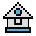Home

# ON ... GOSUB and ON ... GOTO Statements

#### Purpose:

To branch to one of several specified line numbers, depending on the value returned when an expression is evaluated.

#### Syntax:

```ON expression GOTO line numbers
ON expression GOSUB line numbers```

In the ON ... GOTO statement, the value of expression determines which line number in the list will be used for branching. For example, if the value is 3, the third line number in the list will be destination of the branch. If the value is a non-integer, the fractional portion is rounded.

In the ON ... GOSUB statement, each line number in the list must be the first line number of a subroutine.

If the value of expression is zero or greater than the number of items in the list (but less than or equal to 255), GW-BASIC continues with the next executable statement.

If the value of expression is negative, or greater than 255, an "Illegal function call" error occurs.

#### Examples:

`100 IF R<1 or R>4 then print "ERROR":END`

If the integer value of R is less than 1, or greater than 4, program execution ends.

`200 ON R GOTO 150,300,320,390`

If R=1, the program goes to line 150.

If R=2, the program branches to line 300 and continues from there. If R=3, the branch will be to line 320, and so on.